# Singular cohomology

<]]>

<]]>

<]]>

<]]>
• ## Definition

Let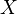be a topological space. Define the singular chain complex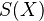. Now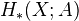and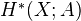is the homology and the cohomology of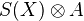and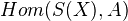, respectively.

This is a bifunctor, contravariant inand covariant in the coefficient group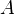.

<]]>

<]]>

<]]>

<]]>

<]]>

<]]>

<]]>

<]]>
• ## Other Information

Completely unverified, from MathOverflow: "Singular cohomology of $X$ can be expressed as sheaf cohomology if $X$ is locally contractible and $F$ is the sheaf of locally constant functions."

<]]>